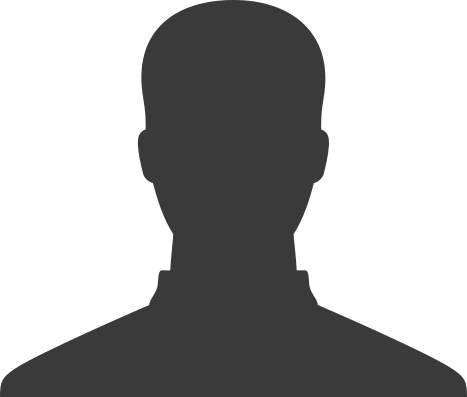# Tutor Subject: Further Maths

A Level Further Maths offers students the opportunity to take the subject a little “further” than A-Level Maths, studying the subject more widely and at a deeper level. (It is not possible to study Further Maths without Maths). This is hard work, but extremely rewarding, and if you are keen to pursue the study of maths beyond school to university, it is highly recommended. If not, and you can study four A Levels you should consider it, but otherwise, universities prefer a balance of A levels (Maths, Further Maths + one other may not be broad enough) Examples of ways in which Further Maths extends ideas are: The solution of quadratic equations by can be extended into the theory of complex numbers (numbers with a real and imaginary part) The concept of sequences first learned in Year 9, is first extended to Arithmetic (successive terms have a common difference) and Geometric series (successive terms are in a common ratio) in A-Level maths and then extended further in Further Maths into techniques for summation of a much wider range of series. The coordinates you are used to are Cartesian coordinates. Further Maths introduces polar coordinates to describe a position on a plane (or even in 3D space) in a different way. Vectors are introduced at GCSE level, then extended to consider vector equations of lines and planes, the angles between them, reflections in them. Hyperbolic functions are studied; these interesting functions in some ways mirror trigonometric functions (sine, cosine) but also have other interesting and useful properties. Further maths also introduces other completely new ideas such as Matrix Algebra using arrays of numbers. Time is also spent studying more applications of maths. There is some choice possible: extending statistics and probability, or mechanics. Decision maths, the maths of algorithms, is another possibility. As with A Level maths course, the overarching theme of the whole course is problem-solving and intertwining the various threads of the course together.## Featured Tutor: Sam

Location: Dereham and also offers Skype tuition

I have been tutoring for a living since graduating from the University of Warwick in 2011. Physics was my degree although I have focused on maths tutoring mainly. I did double maths at A level and got a very good result at maths GCSE (95%). I love tutoring because it gives me the opportunity to …## Featured Tutor: Frances

Subjects: Further Maths Maths
Location: Burnham Market

I have been a successful teacher of maths in a state secondary school in Central Bedfordshire since 2008. I have taught GCSE, A level Maths and Further Maths, all to the most recent specifications I love maths myself and love teaching it. My students, of all abilities, often comment that I explain topics in a …## Featured Tutor: Richard

Location: Dereham, Hockering

He has a BSc in Electrical Engineering from Imperial College London. Richard is a Chartered Engineer (now retired) and has been working with Norfolk Tutors since March 1987.## Further Maths Tutors

In addition to the featured tutors, we have more tutors who teach this subject. Here is the full range of levels we can offer:

GCSE KS4 – Upper secondary KS5 – Sixth Form